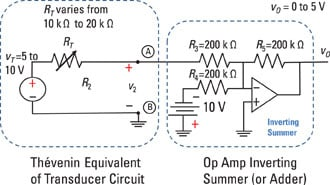##### Circuit Analysis For DummiesHere’s your chance to convert light into electricity using simple operational circuits. You can apply a similar approach to develop instruments that measure other physical variables in the environment, such as temperature and pressure.

You use an input transducer to turn a physical variable into an electrical variable. A photoresistor is an input transducer that converts light energy into a change in resistance, resulting in a change in the current flowing in the circuit. The light is, in fact, an electrical signal.

Suppose you’re dealing with a photoresistor that has a resistance value between 20 MW in total darkness and 20 kW in bright light. If the photoresistor is a linear device, then doubling the amount of light doubles the amount of voltage. You can therefore model a photoresistor as a variable resistor that changes resistance according to the amount of light.

The following figure shows a photoresistor and a complete design of an operational-amplifier (op-amp) circuit to produce an output voltage vO.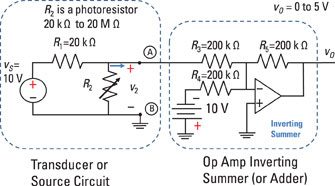Use circuit analysis to show that the op-amp output voltage vO is 0 volts in total darkness and 5 volts in bright light. In other words, show that the voltage range of the output varies from 0 to 5 volts. Here’s how:

1. Determine the output voltage v2 from the transducer.

To determine the range of the output v2 from the transducer (that is, the voltage across Terminals A and B), you can use the voltage divider equation. This equation sets the output voltage equal to the input voltage multiplied by the ratio of the resistance of the output device (R2) to the total series resistance (R1 + R2):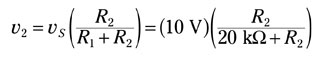2. Determine the lower bound of v2.

The lower bound of the output voltage v2 occurs in bright light, when the photoresistor’s resistance is at a minimum. When R2 = 20 kW, the lower voltage v2L is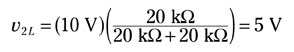3. Determine the upper bound of v2.

The upper bound of the output voltage v2 occurs when the photoresistor’s resistance is highest. In total darkness, R2 = 20 MW = 20,000 kW , so the upper voltage v2U is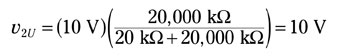The voltage of v2 ranges from 5 to 10 volts.

4. Simplify the transducer (the source circuit) using the Thévenin technique.

The Thévenin technique reduces a source circuit to one single resistor RT and one single voltage source vT. By using the Thévenin equivalent to simplify the transducer, you get a Thévenin voltage vT that varies from 5 to 10 volts and a Thévenin resistance RT that varies from 10 kW to 20 kW.

5. Analyze the op-amp circuit (inverting summer).

The op-amp circuit in the following figure is a typical configuration of an inverting op-amp summer circuit. In this circuit, you have two inputs: one coming from the transducer and another coming from a voltage source of –10 volts. The equation for the inverting operational amplifier is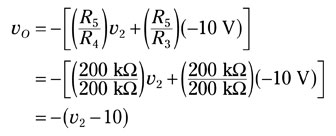Because the voltage range of v2 varies between 5 and 10 volts, the output voltage range of the inverting summer goes from 0 to 5 volts.